# How to Calculate Angle in Degrees?

• Last Updated : 23 Feb, 2022

Angle is measured in degrees (°) and radians. It is formed between the two adjacent sides of a polygon. Every polygon has different sides and different numbers of angles. The formula to find the angles in degrees is useful in geometry and trigonometry. It is essential to understand other concepts of mathematics, such as arc, a central angle of the circle, etc.

1. A full circle = 360°
2. A straight line = 180°
3. A semicircle= 180°
4. A Quarter circle = 90°

### Calculating angles in degrees

There are three different methods of finding angles in degrees, which are as follows:

1. Using the protector D
2. Using the Pythagoras theorem and trigonometry function ins a right angle triangle
3. Using the sum of angles formula
4. Central angle of a circle

### Using the protector D

A protector is a type of ruler or scale that is used to measure distance in centimeters or millimeters. The protector used to measure angles is in a shape of ‘D’ with the value of angles marked from 0 to 180 ° from either direction (right or left).  We need to align the axis with the line on the D to measure the angle. The middle circle of the protector is aligned to the vertex of the angle being measured. The rays along the vertex of angle will help to find the angle in degrees.

### Using the Pythagoras theorem and trigonometry function in a right angle triangle

In trigonometry, there are six functions, sine, cos, cosec, tan, cot, and sec. A right-angle triangle has three sides, base, perpendicular, and hypotenuse.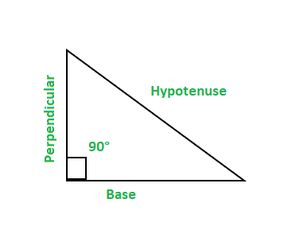• Base: It is an adjacent side to the angle of 90°.
• Perpendicular: It is also an adjacent side to the angle of 90°.
• Hypotenuse: It is a side opposite to the angle of 90°.

A right-angle triangle is represented by an angle of 90° as one of the angles. The total sum of the angle of a triangle is 180°.

• Cosecθ: It is represented as hypotenuse divided by perpendicular.

Cosecθ =• Cotθ: It is represented as base divided by perpendicular.

Cotθ =The other trigonometric functions are represented as:

sinθ =Cosθ =tanθ =secθ =Cosecθ can also be represented as 1/ sinθ

secθ can also be represented as 1/ cosθ

Cotθ can also be represented as 1/ tanθ

Where,

Θ is the angle

Pythagoras Theorem

If two sides of a right angle are known, we can easily calculate the third side of a right angle triangle. In a right-angled triangle, Pythagoras theorem is given by:

(Hypotenuse)2 = (Base)2 + (perpendicular)2

### Sum of angles formula

The Sum of angles refers to the total sum of internal angles of a polygon forming between the two sides. If there are six sides of a polygon, there are around six angles. It helps to find an angle if other angles and the sum of angles of a polygon is known.

The formula to find the total sum of angles of a polygon is given by:

Total sum of angles = 180 (n – 2)

Where,

n is the number of sides of a polygon

Example:

• If n = 4,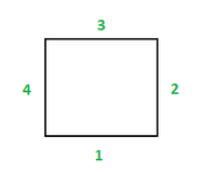Total sum of angles = 180 (4 – 2)

= 180 (2)

= 360 °

If n = 5,

Total sum of angles = 180 (5 – 2)

= 180 (3)

= 540 °

• If n = 6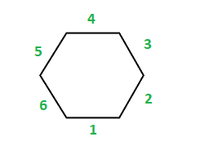Total sum of angles = 180 (6 – 2)

= 180 (4)

= 720°

### Central angle of a circle

A circle is a round shape figure whose boundary is equidistant from its center point. The distance between the center point and the boundary is known as the radius of the circle. The angle formed by the two radii of the circle is known as the central angle. The value of the central angle of a circle lies between 0 and 360 degrees.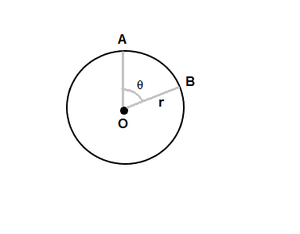The formula to calculate the center angle of a circle is given by:

Length of arc = 2πr × (θ/360)

Θ = 360L/2πr

Where,

r is the radius of the circle

AB is the arc

Theta is the angle in degrees.

L = Arc length

### Sample Problems

Question 1: Find the central angle of a circle of radius 2m with an arc length of 4m?

Solution

The formula to calculate the center angle of a circle is given by:

Θ = 360L/2πr

Where,

r is the radius of the circle

Theta is the angle in degrees.

L = Arc length

Θ = Angle in degrees

r = 2m

L = 4m

Θ = 360 × 4 /2× π × 2

Θ = 114.6°

Thus the central angle of the circle is 114.6°.

Question 2: Find the central angle of a circle of radius 10cm with an arc length of 18cm?

Solution

The formula to calculate the center angle of a circle is given by:

Θ = 360L/2πr

Where,

r is the radius of the circle

Theta is the angle in degrees.

L = Arc length

r = 10cm

L = 18cm

Θ = Angle in degrees

Θ = 360 × 18 /2 × π × 10

Θ = 103.13°

Thus the central angle of the circle is 103.13°.

Question 3: Find the angle of a parallelogram if the other three angles are 80°, 95°, and 105°?

Solution

There are four sides in a parallelogram with the total sum of angles 360°.

Formula to find the sum of angles = 180 (n – 2)

Where,

n is the number of sides of a polygon

Here, n = 4,

The total sum of angles = 180 (4 – 2)

= 180 (2)

= 360 °

Total sum = Angle 1 + Angle 2 + Angle 3 + Angle 4

360 = 80+ 95+ 105+ Angle 4

360 = 280 + Angle 4

Angle 4 = 360 – 280

Angle 4 = 80°

Question 4: Find angle A in the given figure.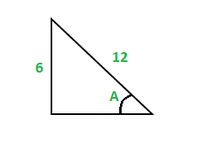Solution:

Given: Hypotenuse = 12

Perpendicular = 6

The trigonometry function to calculate the angle is given by:

sinA = 6/12

A = 30°

Question 5: Find angle A in the given figure.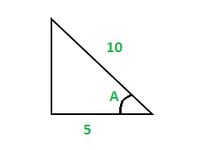Solution:

Given: Hypotenuse = 10

Base= 5

The trigonometry function to calculate the angle is given by:

CosA = 5/10

A = 60°

Question 6: Find the angle of a pentagon if other four angles are 115°, 100°, 105°, and 100°?

Solution

There are five sides in a pentagon with the total sum of angles 540°.

Formula to find the sum of angles = 180 (n – 2)

Where,

n is the number of sides of a polygon

Here, n = 5,

Total sum of angles = 180 (5 – 2)

= 180 (3)

= 540°

Total sum = Angle 1 + Angle 2 + Angle 3 + Angle 4 + Angle 5

540 = 115° + 100° + 105°+100° + Angle 5

540 = 420 + Angle 5

Angle 5 = 540 – 420

Angle 5 = 120°

Question 7:  Find angle A in the given figure.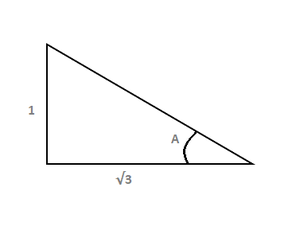Solution:

Given: Base = √3

Perpendicular= 1

The trigonometry function to calculate the angle is given by:

tanθ =tanθ = 1/√3

A = 30°

Question 8: Find the angle of a parallelogram if other three angles are 100°, 70° and 80°?

Solution:

There are four sides in a parallelogram with the total sum of angles 360°.

Formula to find the sum of angles = 180 (n – 2)

Where,

n is the number of sides of a polygon

Here, n = 4,

Total sum of angles = 180 (4 – 2)

= 180 (2)

= 360°

Total sum = Angle 1 + Angle 2 + Angle 3 + Angle 4

360 = 100 + 70 + 80 + Angle 4

360 = 250 + Angle 4

Angle 4 = 360 – 250

Angle 4 = 110°

Thus, the other angle is 110°.

Question 9: Find the angle of a hexagon if other five angles are 120°, 115°, 110°, 125°, and 105°?

Solution

There are six sides in a hexagon with the total sum of angles 720°.

Formula to find the sum of angles = 180 (6 – 2)

Where,

n is the number of sides of a polygon

Here, n = 6,

Total sum of angles = 180 (6 – 2)

= 180 (4)

= 720°

Total sum = Angle 1 + Angle 2 + Angle 3 + Angle 4 + Angle 5 + Angle 6

720 = 120 + 115 + 110 + 125 + 105 + Angle 6

720 = 575 + Angle 6

Angle 6 = 720 – 575

Angle 6 = 145°

Thus, the sixth angle of hexagon is 145°.

My Personal Notes arrow_drop_up